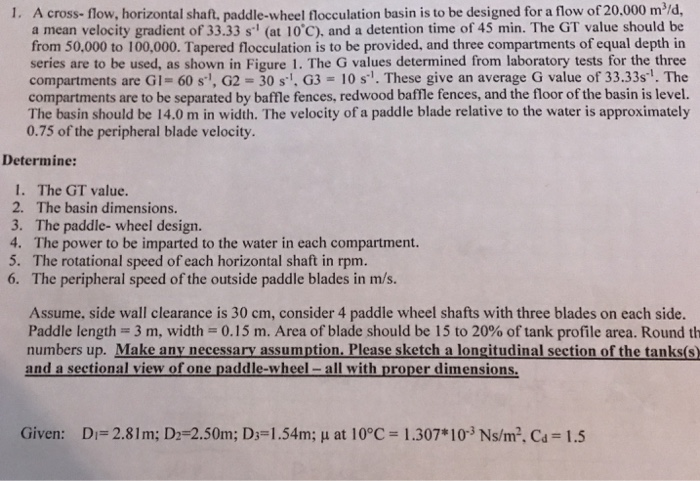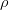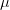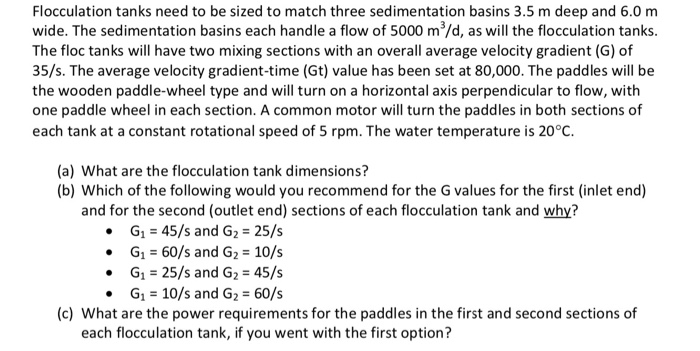# 1. A cross- flow, horizontal shaft, paddle-wheel flocculation basin is to be designed for a flow...Ans) Given,

Q = 20000 m3 /day or 0.231 m3 /sec

Mean velocity gradient (G) = 33.33 /sec

Detention time(t) =45 min or 2700 sec

G1 = 60/sec , G2 = 30/sec , G3 = 10/sec

Width of basin = 14 m

1) GT value

GT = G x t

= 33.33 x 2700

= 89991

Since the GT value is between 50000 to 100000, therefore the detention time is satisfactory

2) Basin dimensions

Basin volume(V') = flow x detention time

= 0.231 x 2700

= 624 m3

Profile area = Volume/ width

= 624/14

= 44.57 m2

Assuming square compartments, and length as thrice of its depth(d),

therefore, d x 14 x 3d = 624

d2 = 14.86

or d = 3.85 m

Hence, length(L) = 3 x 3.85

= 11.55 m

3) P = Cd x A xx (v3 /2)

where, Cd = Coefficient of drag= density of liquid

v = velocity of paddle relative to water

P = 1.5 x (3 x 0.15) x 1000 x ( v3/2)

Peripherial velocity(V) = RPM x d2 x/ 60

Assuming Rotation of paddle wheel as 4 RPM,

V= 4 x (2.50 x 4) x 3.141 /60

V = 2.09 mps

Therefore, v = 0.75 x 2.09

= 1.57 mps

Therefore, P = 1.5 x 3 x 0.15 x 1000 x 1.573 / 2

= 1306.08 W

4) Power to be imparted (P') to the water in each compartment

P' =G2 V'

where, V' = basin volume= dynamic viscosity of water (0.00131 N -s/m2)

G = velocity gradient for each compartment

P' ( for first compartment) = 0.00131 x 602 x 624

= 2920 W

P'(for second compartment) = 0.00131 x 30 x 30 x 624

= 736 W

P' (for third compartment) = 0.00131 x 10 x 10 x 624

= 82 W

#### Earn Coin

Coins can be redeemed for fabulous gifts.

Similar Homework Help Questions
• ### Horizontal-shaft paddle wheel ﬂocculators are to be used for ﬂocculation in a water plant with a...

Horizontal-shaft paddle wheel ﬂocculators are to be used for ﬂocculation in a water plant with a design ﬂow rate of 150 mld (million-liter per day) and water temperature of 10oC. Flocculation is to be designed with two parallel trains and each train is to be made off ﬁve stages of ﬂocculation in series. The total detention time for ﬂocculation is to be 20 min. Two paddle wheels will be on the shaft in each stage. The paddle wheel design contains...

• ### ENV Water Supply Flocculation tanks need to be sized to match three sedimentation basins 3.5 m...ENV Water Supply Flocculation tanks need to be sized to match three sedimentation basins 3.5 m deep and 6.0 m wide. The sedimentation basins each handle a flow of 5000 m/d, as will the flocculation tanks. The floc tanks will have two mixing sections with an overall average velocity gradient (G) of 35/s. The average velocity gradient-time (Gt) value has been set at 80,000. The paddles will be the wooden paddle-wheel type and will turn on a horizontal axis perpendicular...